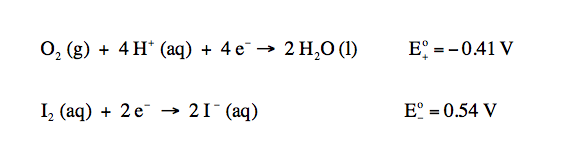Clutch Prep is now a part of Pearson
Ch.16 - Electroanalytical TechniquesWorksheetSee all chapters

# Fundamentals of Electrolysis

See all sections
Sections
Fundamentals of Electrolysis
Gravimetric Analysis
Coulometry
Voltammetry
###### Electrolysis

Concept #1:

Electrolysis deals with passing an electrical current through a substance in order to produce chemical changes.

###### Expert Q&A

Ask unlimited questions and get expert help right away.

Example #1:

Aluminum can be electroplated at the cathode of an electrolysis cell by the half-reaction:

Al3+ (aq) + 3 e  → Al (s)

How much time would it take for 825 mg of aluminum to be plated at a current of 4.1 A?

###### Expert Q&A

Ask unlimited questions and get expert help right away.

Example #2: In the electrolysis of molecular iodine to iodide ions for 0.15 M NaI solution containing 4.2 x 10-4 M I2 at a pH = 6.00 with PO2 = 1.25 bar, calculate the voltage needed to drive the reaction.

###### Expert Q&A

Ask unlimited questions and get expert help right away.Practice: During electrolysis the concentration of I2 increases to 8.3 x 10 -3 M, while all other concentrations remain unchanged. If the electrical resistance is 1.8 ohms, the current is 71 mA, the anode overpotential is 0.013 V and the cathode overpotential is 0.115 V, what is the voltage needed?

###### Electrolysis Calculations

Example #3:

Gold can be plated out of a solution containing Au3+ based on the following half reaction:

Au3+ (aq) + 3 e  → Au (s)

a) What mass of gold is plated by a 41 minute flow of 6.8 A current?

###### Expert Q&A

Ask unlimited questions and get expert help right away.

Example #4:

A solution of Mn+5 is used to plate out Mn in an electrochemical cell. If a total of 1.13 g of Mn is plated out in a total time of 1600 seconds, what was the electrical current used? (MW of Mn is 54.94 g/mol)

###### Expert Q&A

Ask unlimited questions and get expert help right away.

Example #5:

EXAMPLE: If steady current of 15 amperes is provided by a stable voltage of 12 Volts for 600 seconds, answer each of the following questions.

a)  Calculate the total charge that passes through the circuit in this time.

b)  Calculate the total number of moles of electrons that pass through the circuit in this time.

c)  Calculate the total amount of energy that passes through the circuit in this time.

d)  Calculate the power that the battery provides during this process.

###### Expert Q&A

Ask unlimited questions and get expert help right away.

###### Expert Q&A

Ask unlimited questions and get expert help right away.